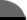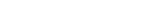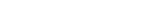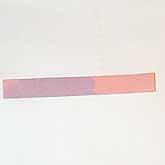Facts By Category:» Physics  » Astronomy  » Chemistry  » Biology  » Mathematics  » Geology  » Engineering  » Medicine  » Science  ScienceIQ Team:»Writers & EditorsxUmp.com
Science Supplies,

Physics & Astronomy
Online
What Is pH?Anyone who is the least bit familiar with vinegar, nausea, sodium bicarbonate, and ammonia-based cleaning solutions probably has a very good 'feel' for the different natures of acidic and basic solutions. But what the heck is 'pH' all about? To understand that one first has to know what makes a solution acidic or basic. The water molecule, H2O (or H-OH) can separate into H+ (hydrogen ion, or hydronium ion when dissolved in water), and OH- (hydroxide ion). When this happens, equal quantities of H+ and OH- are produced and the solution remains neutral. But since acidic and basic solutions are most decidedly not neutral, something else is involved in those cases. Materials that act to increase the amount of H+ relative to OH- in a solution are called acids, while those that act to increase the relative amount of OH- in a solution are called bases.

To get to a good understanding of pH, one has also to understand the concept of the 'mole' (not the rodent, but the material quantity...). A mole of any substance is defined as an amount of that substance whose weight in grams has the equivalent value of the molecular or atomic weight of that substance. For example, the compound known as water, H2O , has a molecular weight of 18 atomic mass units; one mole of water therefore weighs 18 grams. The mole concept allows us to define concentrations of materials in solutions in terms of 'molarity', the number of moles of a particular material in each liter of the solution. The pH scale allows us to describe acid and base concentrations in an accurate manner, and to carry out concentration change calculations very easily.

Whatever the concentration of H+ ions in the solution, the pH is just the negative value of the base 10 logarithm of that concentration. In neutral water, the [H+] is 0.0000001, or 10-7 Molar, so the pH is 7. Now suppose we were to add a small amount of an acid such as hydrochloric acid, HCl; just enough to make the H+ concentration 0.000001, or 10-6 Molar. The pH would now be 6, and there would be ten times more H+ in the solution, and it is therefore ten times more acidic than water. Clearly, the smaller the pH value, the more acidic is the solution. Basic solutions work the same way, only backwards. Suppose a small amount of a basic material were to be added to neutral water; just enough to leave only one-tenth of the H+ ions. The [H+] would now be 10-8 Molar, and the pH would be 8. Adding more OH- decreases the [H+] accordingly. Thus the higher is the pH value, the more basic is the solution.Richard M. J. Renneboog, MS Richard M. J. Renneboog is an independent private technical consultant and writer in both chemical and computer applications. Endeavors have included preparation of scripts for instructional and promotional video, corporate website design, curriculum development for training in advanced composites technology, and development.Acid-Base, Fluids, and Electrolytes Made Ridiculously Simple (MedMaster Series)by Richard A. Preston
 Home | Privacy Policy | Cookie PolicyCopyright © 2002-2020 ScienceIQ.com - All Rights Reserved